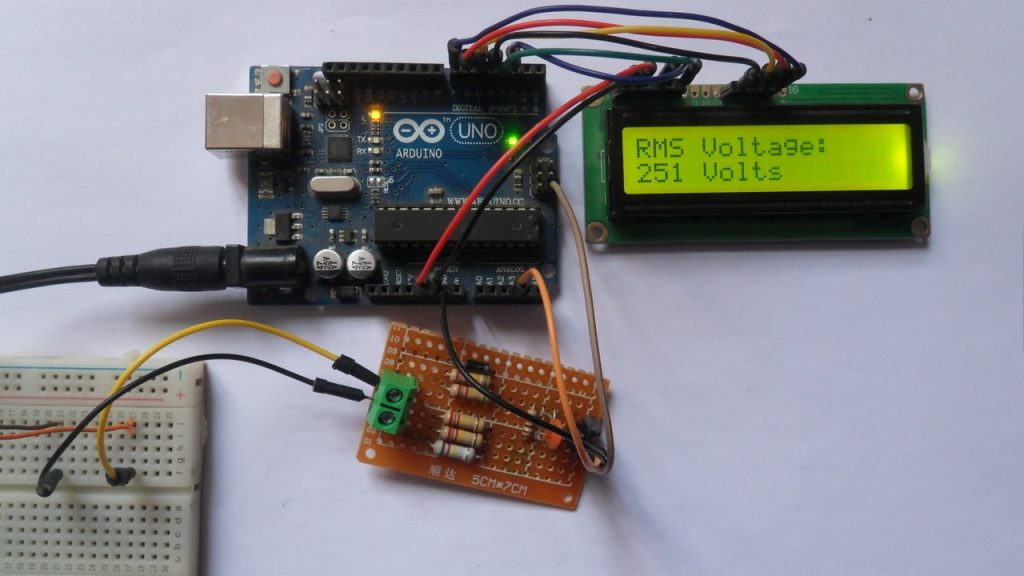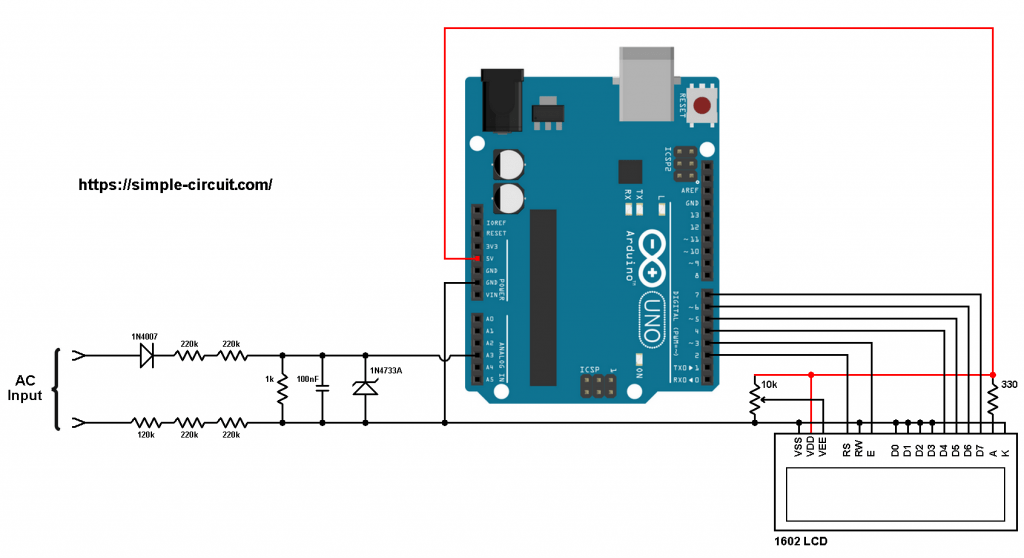# Measure AC Voltage with Arduino – AC Voltmeter

This post shows how to build a simple AC voltmeter that measure AC voltage with Arduino UNO board where voltage value is printed on 16×2 LCD screen.
This voltmeter can easily measure 110/220/380V AC voltages with frequency of 50 or 60Hz.

No warranty is provided with this project, do it at your own risk!

Hardware Required:
This is a list of all components required to build this project.

• Arduino UNO board   —-> Atmega328P datasheet
• 16×2 LCD screen
• 330 ohm resistor
• 10k ohm variable resistor or potentiometer
• 4 x 220k ohm resistor
• 120k ohm resistor
• 1k ohm
• 100nF ceramic capacitor
• 1N4733A 5.1V Zener diode (or equivalent)
• 1N4007 diode (or equivalent)
• Jumper wiresArduino AC voltmeter circuit:
The image below shows project circuit diagram.The AC input is connected to the circuit as shown where diode 1N4007 is used to eliminate the negative half cycles.
After we get the positive parts of the AC voltage signal, it enters to a voltage divider because the Arduino UNO board can’t deal with voltages higher than 5V. The voltage divider is composed of 4 x 220k ohm resistors, 1 x 120k ohm resistor and 1 x 1k ohm resistor, so the impedance of this voltmeter is about 1M ohm (mega-ohm).

The Arduino reads the voltage across the 1k ohm resistor which is equal to the input voltage divided by 1001k ohm (voltage divider equation).
So, if the input voltage is 220V then the voltage across the 1k ohm resistor is 0.220V = 220mV (RMS values).

The 100nF ceramic capacitor is used to stabilize and eliminate noise of voltage signal across the 1k resistor. The 1N4733A Zener diode (reverse-breakdown voltage is 5.1V) is used to protect the Arduino board from voltages higher than 5.1V.

The 16×2 LCD screen (2 rows and 16 columns) is used to display the value of the input voltage where:
RS —> Arduino digital pin 2
E   —> Arduino digital pin 3
D4 —> Arduino digital pin 4
D5 —> Arduino digital pin 5
D6 —> Arduino digital pin 6
D7 —> Arduino digital pin 7
VSS, RW, D0, D1, D2, D3 and K are connected to Arduino GND,
VEE to the 10k ohm variable resistor (or potentiometer) output,
VDD to Arduino 5V and A to Arduino 5V through 330 ohm resistor.

VEE pin is used to control the contrast of the LCD. A (anode) and K (cathode) are the back light LED pins.

Arduino AC voltmeter code:
The following Arduino code measures the RMS value of the input AC voltage by detecting the maximum value of the half wave and then divide it by square root of 2 (√2).
So: Vrms = Vmax/√2

The Arduino detects the maximum value by reading analog voltage on channel 3 (A3) multiple times.
The function get_max() reads channel A3 voltage 100 times during period of more than 20ms (because for frequency of 50Hz the period is 20ms).

In this project the Arduino actually doesn’t give the True RMS of the input AC voltage because it uses the function: Vrms = Vmax/√2
That means the Arduino will give a correct measurement for a sine wave AC voltage input only!

Full Arduino code:

The video below shows a protoboard circuit of the project:

And this one shows Proteus simulation:

Proteus simulation file download (use Proteus version 8.8 or higher to open it!):
Arduino AC voltmeter

1.djalltra says:

great tutorial can you please do ac ahmeter project

2.Antony says:

Hi
Thanks for this toturial… great work.
I followed it but without the lcd . i wanted to check the voltage from serial monitor.
the problem is that if use a multi-meter and take readings in the two ends of 1k resistor everything seems to work… when i am connecting it as you showing in the drawing to arduino ground then i can get no readings … it become zero
arduino monitors shows also zero

3.Marc Poelmans says:

when you connect the usb port, the ground from your pc short circuits one of the tho inputs from the arduino because there is no galvanic isolation from the mains to your arduino. the shield on the USB is also the mains Ground

1.Simple Projects says:

Yes you shouldn’t connect the Arduino to PC! The Arduino should be powered from another source (for example external battery).
Actually there’ll be no short circuit because there’s a resistor of 560k between the ground and mains line.

4.bash says:

thank u for your great work. please when i try using Arduino mega to run the code it does not run. any help

5.malik says:

i tried it 12c lcd, but in my case lcd shows the value 777 Volts even no pin connected to the A3, i change the pin to A1, but the result is the same

6.Uku says:

Hello,

Thank you for the project. What is the blue-lcd device part no. that you are demonstrating at the end of the video?

7.Gabs says:

What if I have an Arduino Mega? The “INTERNAL” configuration is not avaible in this product

1.Gabs says:

Nevermind, for Mega is “INTERNAL1V1”

8.Gabs says:

The other problem that I have is that when the circuit is not conected to the source, it still shows me values not equal to 0 on the LCD screen

9.sunnyverma1984 says:

How to check wave form of AC and plot it on screen

10.Dammy says:

Thanks a lot. I followed the tutorial and it worked fine. Why did you use 600 (Amplitude) for Vsine? I’m aware that the AC Frequency is 50Hz.

Thanks.

This site uses Akismet to reduce spam. Learn how your comment data is processed.